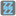• # FixxBuzz AssertionError: 15 is divisible by 3 and

## Question related to missionFizz Buzz

i'm running the following code and getting error 'AssertionError: 15 is divisible by 3 and 5'. Don't know where i made a mistake.

def checkio(number): if ((number%3==0) and (number%5==0)): return "FixxBuzz" elif (number%3)==0: return "Fizz" elif (number%5)==0: return "Buzz" else: return str(number)

if name == 'main': assert checkio(15) == "Fizz Buzz", "15 is divisible by 3 and 5" assert checkio(6) == "Fizz", "6 is divisible by 3" assert checkio(5) == "Buzz", "5 is divisible by 5" assert checkio(7) == "7", "7 is not divisible by 3 or 5" print("Coding complete? Click 'Check' to review your tests and earn cool rewards!")8